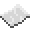# math.randomseed

Sets the seed for the random number generator used by math.random. The same seeds produce will always produce the same random numbers.ExampleGenerate two sequences with the same seed
Print two sequences of randomly generated numbers using the same seed
Code
```<nowiki>
math.randomseed(1234)
print("First: " .. math.random())

math.randomseed(1234)
print("Second: " .. math.random())
</nowiki>
```
Output First: 0.12414929654836
Second: 0.12414929654836

math.randomseed
Function
Syntax
math.randomseed(
• seed : number
)

Returns nil
API math
Source Lua (source)